##Effective Altruism ForumEA Forum

Cause prioritization

# 7

This is the technical guide to the Causal Networks Model, created by CEA summer research fellows Alex Barry and Denise Melchin. Owen Cotton-Barratt provided the original idea, which was further developed by Max Dalton. Both, along with Stefan Schubert, provided comments and feedback throughout the process.

This is of a multipart series of posts explaining what the model is, how it works and our findings. We recommend you read the ‘Introduction & user guide’ post first, and only read this if you are interested in the technical details of how the model works. The structure of the series is as follows:

1. Introduction & user guide (Recommended before reading this post)

2. Technical guide (this post, optional)

3. Findings (writeup of all findings)

4. Climate catastrophe (one particularly major finding)

The structure of this post is as follows:

1. Introduction to elasticities and differentials

2. Explanation of how elasticities and differentials are used in the model

3. Explanation of how the model is technically implemented

## 1 . Introduction to elasticities and differentials

As set out in Part 1, the quantitative model we created is designed to allow us to compare the indirect effects of different common EA activities. At its core, the model is based on elasticities and differentials, which are ways different variables can relate to each other:

Elasticities: The percentage change in B has a linear relationship to the percentage change in A. For example, each 1% increase in A causes an x% change in B for some fixed x (so a 2% increase in A causes a 2x% change in B, and so on).

Differential: The actual change in B has a linear relationship to the actual change in A. For example, increasing A by 1 unit increases B by x units for some fixed x (so increasing A by 2 would increase B by 2x, and so on).

## 2. How elasticities and differentials are used in the model

The model is built around a collection of nodes, each of which is considered as either an elasticity or a differential. Some of the nodes are connected together, and nodes which are connected influence each other in the manner described above.

Now let's look at a simple example with differentials, considering the relationship between donations to AMF and lives saved. The most basic model would be:Here, donating to AMF leads directly to saving lives. We could describe this situation with a differential, e.g. each \$1,800 donated to AMF saves one life (or each dollar donated saves 1/1,800 lives). To illustrate this we could add this weighting to the connection:To look at the situation in more detail we could add another node, ‘Bednets distributed’ (assuming all of the lives AMF saves are by it distributing bednets). We can then break down AMF’s \$1,800 to save a life into every \$3 distributing a bednet, and every 600 bednets distributed saving a life:So although funding AMF does save lives indirectly, here the nodes are not directly connected as the effect is entirely mediated by the bednets node.

Whilst representing the connections between nodes with graphs like this is fine for small cases, it gets harder to keep track of as the number of nodes increases . An alternative approach is to list all the connections in a table (i.e. a matrix), making in our case: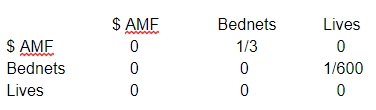The way to interpret the matrix is that everything a node is directly connected to can be seen by looking along its row (along with their weightings) and everything that is directly connected to a node can be seen by looking in that node’s column. So in this case looking in the AMF row tells us that funding AMF by \$1 increases the number of bednets distributed by ⅓, but has no other direct effect. Looking at the lives saved column tells us that only bednets directly affects lives saved.

We can do something similar for the elasticities case, for example by looking at how the size of the EA community affects both the number of EAs in government and AMF funding.

In this case we think that some constant percentage of EAs are in government, so increasing the number of EAs by 1% also increases the number of EAs in government by 1%. However, we assume that only half of the funding for AMF comes from EAs, so increasing the number of EAs by 1% would only increase AMF’s funding by 0.5%.

In graph form:Now in this case, the weightings over the connections represent elasticities instead of differentials. Therefore, instead of saying that increasing EA movement size by 1 increases the number of EAs in government by 1, this instead shows that increasing EA movement size by 1% increases the number of EAs in government by 1%. (And increasing EA movement size by 1% increases AMF funding by 0.5%)

Again this can be represented by a matrix with the same format as before: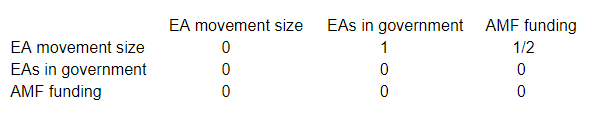When designing our model, we made the decision to always model some nodes as elasticities, and others as differentials. So connections to a ‘differential node’ must always be calculated in terms of how many additional units of that node they cause, and similarly connections to an ‘elasticity node’ must always be in terms of the percentage change caused to that node.

Here is an example to make this clearer. Say A is an elasticity node and B a differential node. Then the A -> B connection is of this form: a 1% increase in A increases B by x units. Meanwhile the B -> A connection is of this form: increasing B by 1 unit changes A by y%.

As the weightings of connections can represent different things depending on the kinds of nodes they connect, we will keep track of which node is which, marking nodes with an E or D as appropriate.

Here is a more general example, featuring both elasticities and differentials:Here the connection between AMF funding and bednets needs to represent the number of additional bednets distributed as a result of increasing AMFs funding by 1%. AMF distributed roughly 12,000,000 bednets in 2016, and spends all of its budget on this, so increasing funding by 1% will lead to roughly 120,000 more bednets being distributed.

Again we can represent this in matrix form, with an additional column on the left keeping track of which nodes are differentials and which are elasticities.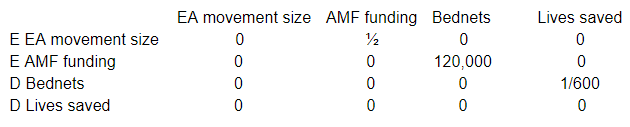So far we have just looked at direct effects. How does the model account for indirect effects? The theory is that although our model does not explicitly include a time element, we still want a way for changes to ‘propagate’. Returning to the AMF -> bednets -> lives saved example:The obvious thing to do is keep following the arrows from the node we started at, multiplying by the weighting of each connection as we go. So we would start by funding AMF by, say, \$900, and then follow the arrow to the Bednets node, multiplying by ⅓ as we go to get 300 additional distributed bednets. We then continue along the next arrow to the lives saved node, multiplying by 1/600 to get a result of ½ of a live saved, which fits with our assumption that AMF saves lives for \$1,800.But how can we come up with an process equivalent to this for the matrix case? While we could manually repeat the previous method of trying to imagine funding starting from AMF and follow the connections until we hit the end, it turns out that there is an easier way.

We consider starting by increasing the funding for AMF by \$1,800. This can be represented as a (row) vector of our starting changes (1800, 0, 0) where the first, second and third entries represent the initial change in AMF funding, bednets, and lives saved respectively. So as we start by just donating money, and don’t give out any bednets or save any lives ourselves, the second and third entries are zero.

Right-multiplying this vector by the matrix of connections above then gives: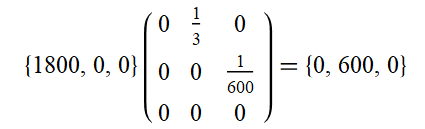This can be interpreted as an increase in the funding to AMF leading to an increase in the number of bednets. Note that as AMF funding does not lead to any ‘new’ AMF funding (in our toy model) the corresponding entry in the vector is now zero.

We can now repeat this process again, multiplying: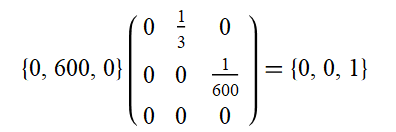This again can be interpreted as the 600 addition bednets causing 1 addition life to be saved. However here’s what happens if we repeat the process again: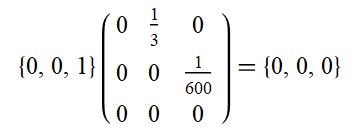So we can see that saving a life has no causal outputs (in our toy model!) and no matter how many times we multiply this final vector by the matrix it will stay at zero.

So we can find the total impact by summing our three non-zero vectors, to get: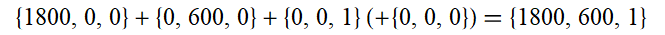Thus the model tells us that increasing AMFs funding by \$1,800 increases AMFs funding by \$1,800 (helpful...), results in 600 additional bednets being distributed, and saves 1 life.

While this might seem a lot more complicated than just following the arrows on the graph, it has the advantage of working in exactly the same way for graphs with many more nodes, letting us scale up easily.

An additional advantage is that this method can also be seen to work in more complicated cases with feedback loops. Consider an elasticities case with only two nodes, EA movement size and EA outreach funding. Let’s say that outreach funding is directly proportional to movement size, but increasing outreach funding by 1% only increases movement size by 0.5%.This can be captured by the following matrix:Increasing outreach funding by 20% can therefore be represented by the starting vector (0, 20). The direct effects of this increase can be computed as follows: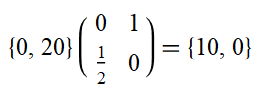The outreach funding leads directly to a 10% increase in movement size. However, going further we can see the following: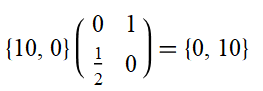This then leads back to a 10% increase in outreach funding, putting us in a similar situation to when we started. Therefore in this case the vector will never hit zero, and instead we can model the overall effects of increasing funding through an infinite sum: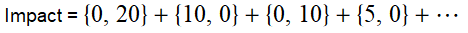To make sense of this it is helpful to rewrite the terms by factoring the matrix back out to get: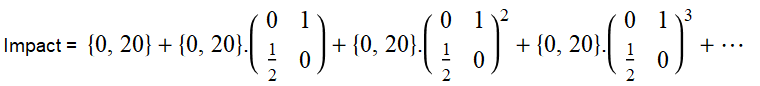We can then factor this again as: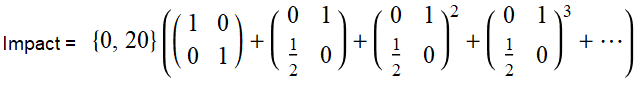Luckily there is a formula for such infinite matrix sums . It states, for a matrix M:

I + M + M^2 + M^3 + ….   = (I - M)^-1 (Where I is the identity matrix)

Therefore we can calculate the exact result, avoiding the infinite sum, and find the following: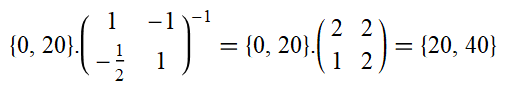So despite the feedback loop meaning that the vectors never hit zero, we can still get a sensible answer. We find that increasing outreach spending by 20% results in 20% greater movement growth (partially by causing an additional 20% to be spent on outreach).

We can also see that we could have applied this approach to the original AMF problem, where the starting vector is (1800, 0 , 0). Remembering the matrix in this case was: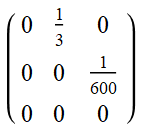This way we get: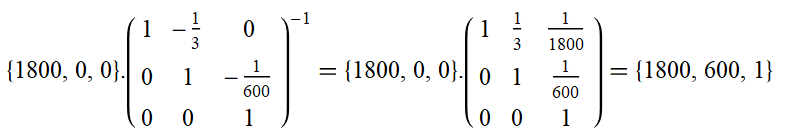Which is what we found initially.

This approach in fact works for any of the kinds of models we have considered, no matter the number of nodes, whether they contain feedback loops (as long as they decay over time) or if they mix elasticities and differentials.

Therefore, given an initial matrix of connections M, the model is linear with the input row vector of changes being right multiplied by the matrix (I - M)^-1 to give the output vector.

## 3. Implementation

The model basically functions as a scaled-up version of the examples given above. You can set the nodes in the ‘matrix’ sheet and fill in the values of the connections weightings there (with blank spaces being interpreted as zero). It can currently handle up to 100 nodes, but this could be easily extended.

The rows and columns are numbered to avoid needing to repeat the names of the nodes as headings for the columns. Thus if row 4 is, for example, AMF funding, then all values in column 4 are connections leading to AMF funding.

Column B keeps track of whether nodes are differentials or elasticities. Conditional formatting inside the matrix then sets cells to different colours to help keep track of the kinds of nodes it is connecting, with colours encoding the following:

Yellow represents elasticity to elasticity (1% change in A causes x% change in B).
Green represents elasticity to differential (1% change in A causes change of x units in B).

Purple represents differential to elasticity (increase of 1 unit in A causes x% change in B).

Blue represents differential to differential (increase of 1 unit in A causes change of x units in B).

Once the nodes and the connections between them are set up, the calculations are done using other sheets:

‘Calc 1’ sheet is used to find I - M (and fill in blank values with zeros).

‘Calc 2’ sheet is used to find (I - M)^-1.

For historical reasons the ‘Effective Matrix’ sheet then finds (I - M)^-1 - I, which is then used for the final calculations. This has very similar properties to (I - M)^-1, but does not count (in the AMF example) funding AMF as producing AMF funding as an output. In practise these effects are then put back in later, so this is just an historical aberration that did not seem worth the time to remove. (In theory all the information of the model is contained within the ‘Effective Matrix’ sheet, as this tells you how the model will react to any given changes in funding, but it is less user friendly than the user tool.)

One can then use the model in the ‘Manual Input’ tab, in which the nodes are copied from the ‘Matrix’ sheet and the input vector is the change column. This is then right matrix multiplied by the effective matrix to produce the result column. There is also the option of filling in the initial values column to get everything converted to actual units instead of a mixture of percentage changes and absolute changes

In general everything should only depend on the ‘Matrix’ sheet, so if you want to customise anything (e.g. change connection weightings, add new nodes) it should generally be possible. (The ‘User Tool’’ sheet is an exception, as it is fine-tuned to the current setup)

‘Calc 3’ is used to do the behind the scenes calculations for the ‘User Tool’ sheet, and it is largely a copy of ‘Manual Input’ with a few additional sections to compute the moral outputs.

--------------------------------------------------------------------------------------

Our next post will be an overview of the findings of the model which constitutes Part III of the series.

Feel free to ask questions in the comment section, or email us (denisemelchin@gmail.com or alexbarry40@gmail.com).

--------------------------------------------------------------------------------------

 Technically point elasticites and point differentials.

 Subject to the some conditions, which in our case are equivalent to requiring feedback loops (such as the one above) to decay over time instead of growing larger.

New Comment